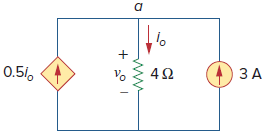# SSC JE Electrical Important MCQ Part 12

SSC JE Electrical Important MCQ PDF Part 12

1.Lenz’s Law follows from

A. Principle of conservation of mass

B. Principle of superposition

C. Principle of conservation of energy

D. Principle of homogeneity

2.The coupling coefficient for the transformer belowA. 0

B. 1

C. 0.56

D. 0.98

3.An ideal transformer, connected to a 240 V mains, supplies power to a 12 V, 150 W lamp.The current drawn from the mains is

A. 0

B. 1

C. 0.05

D. 0.625

(Secondary current I₂=P/V₂=150/12=12.5 A
The transformation ratio n=V₂/V₁=12/240=0.05
The current drawn from supply I₁=I₂×n=12.5×0.05=0.625 A)

4.The dependent source in Fig is(a) Voltage-controlled current source

(b) Voltage-controlled voltage source

(c) Current-controlled voltage source

(d) Current-controlled current source

Answer  : D (A current source that depends on a current input is generally referred to as a Current Controlled Current Source or CCCS)

5.The current iₒ and voltage vₒ in the circuit shown in Fig isA. 6 A,24 V

B. 3 A,12 V

C. 4 A,8 V

D. 2 A,4 V

(Applying KCL to node a, we obtain
3 + 0.5 iₒ = iₒ ⇒ iₒ = 6 A
For the 4-Ω resistor, Ohm’s law gives
vₒ= 4 iₒ = 24 V)

6.What is the form factor of pure sine wave
A. 1.11

B. 1.414

C. 1.57

D. 0.909

Answer  : A (Form Factor is the ratio between the average value and the RMS value and is given as. For a pure sinusoidal waveform the Form Factor will always be equal to 1.11)

7. A network with b branches, n nodes, and l independent loops will satisfy which of the

A. b=l-n+1

B. b= n-l+1

C.b=l+n-1

D. b=l-n-1

8.Total losses occurring in a transformer when it is operating at its maximum efficiency are 2000 W. The total copper losses is

A. 2000 W

B. 1000 W

C. 1500 W

D. 800 W

(The condition for maximum efficiency is, Full load copper loss Pc = Iron loss, Pi ,Total loss P = Pc + Pi = 1000 + 1000 = 2000 W)

9.A salient pole machine is used for

A. Low speed applications in Hydro plants

B. High speed applications in Hydro plants

C. Low speed applications in Thermal plants

D. High speed applications in

10.If a three-phase, 8–pole, 50 Hz induction motor runs at a speed of 735 r.p.m. then the slip is

A. 0.02

B. 0.03

C. 0.04

D. 0.05

11.In an oil- filled transformer, oil is provided for

A. Cooling

B. Insulation

C. Lubrication

D.Both Cooling and insulation

12.Kirchoff’s Laws are applicable for

A. DC circuits only

B. Circuits with rectified AC supply

C. Both AC and DC circuits

D.AC circuits only

13.In a Y-Y system, a line voltage of 220 V produces a phase voltage of

A. 381 V

B. 311 V

C. 220 V

D. 127 V

14.A commutator in a DC machine can convert

A. AC to DC

B. DC to AC

C. Pulsating to DC

D. Both AC to DC & DC to AC

Answer  : D (In DC Generator the commutator converts AC to DC,In DC Motor DC to AC ,Thus Commutator in a DC machine converts both AC to DC and DC to AC)

15.The main purpose of using a core in transformer is to

A. Decrease iron loss

B. Prevent eddy current loss

C. Eliminate magnetic hysteresis

D. Decrease reluctance of the magnetic circuit

16.In a particular meter, the operating torque is directly proportional to the current passing through it , then the type of meter is

A. moving iron

B. moving coil

C. electrostatic

D. induction

17.Short circuit test on transformer is conducted to obtain

A. Core losses at any load

B. Hysteresis loss only

C. Ohmic Loss

D. Eddy Current Loss only

18.Out of the following which machine is also known as a generalized transformer

A. DC motor

B. Induction Motor

C. DC generator

D. Synchronous motor

19.The rating of a transformer is expressed in

A. KVA

B. KW

C. HP

D. W

20.If the current through a 10-mH inductor increases from zero to 2 A, how much energy is stored in the inductor

A. 40 mJ

B. 20 mJ

C. 10 mJ

D. 5 mJ

Answer  : B (Energy is stored in the inductor=LI²/2=10×2²/2=20 mJ)